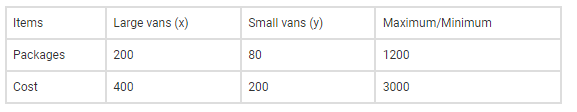# A firm has to transport 1200 packages using large vans

Question:

A firm has to transport 1200 packages using large vans which can carry 200 packages each and small vans which can take 80 packages each. The cost for engaging each large van is Rs 400 and each small van is Rs 200. Not more than Rs 3000 is to be spent on the job and the number of large vans cannot exceed the number of small vans. Formulate this problem as a LPP given that

the objective is to minimize cost.

Solution:

Let’s consider x and y to be the number of large and small vans respectively.

From the given information the below constrains table is constructed:Now, the objective function for minimum cost is

Z = 400x + 200y

Subject to the constrains;

200x + 80y ≥ 1200 ⇒ 5x + 2y ≥ 30 ….. (i)

400x + 200y ≤ 3000 ⇒ 2x + y ≤ 15 ….. (ii)

x ≤ y …. (iii)

and x ≥ 0, y ≥ 0 (non-negative constraints)

Therefore, the required LPP is to minimize Z = 400x + 200y

Subject to the constraints 5x + 2y ≥ 30, 2x + y ≤ 15, x ≤ y and x ≥ 0, y ≥ 0.